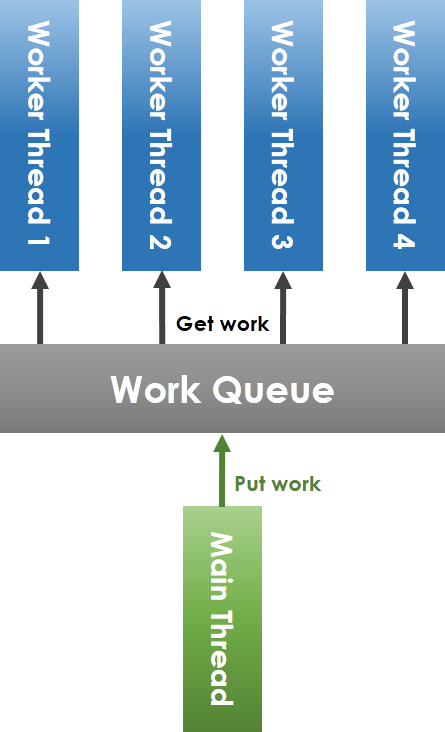#DAY 10
0

## 經典範例

PS：我覺得 C 語言的程式碼，我現在這種寫法的可讀性很低＝＝ Sorry

• 一般的 linked list
``````typedef struct __NODE {
int val;
struct __NODE *next;
} NODE;
``````
• Coarse-Grained Synchronization
``````typedef struct __NODE {
int val;
struct __NODE *next;
} NODE;

typedef struct {
NODE *head;
pthread_mutex_t mutex;
} LIST;
``````

``````void add(NODE *head, int newVal) {
NODE *curr, *pred;
pthread_mutex_lock(mutex);// 在最外面鎖起來

pred = head;
curr = pred->next;
while(curr->val < newVal) {
pred = curr;
curr = curr->next;
}
NODE *new = malloc(sizeof(NODE));
new->val = newVal;
new->next = curr;
pred->next = new;

pthread_mutex_unlock(mutex);
}
``````
• Fine-Grained Synchronization
``````typedef struct __NODE {
int val;
struct __NODE *next;
pthread_mutex_t mutex;
} NODE;
``````

``````void add(NODE *head, int newVal) {
NODE *pred;
pred = head;
pthread_mutex_lock(head->mutex);// 鎖起來

Node *curr = pred->next;
pthread_mutex_lock(curr->mutex);// 連續鎖了兩個
while(curr->val < newVal) {
pthread_mutex_unlock(pred->mutex);// 馬上解放上一層
pred = curr;
curr = curr->next;
pthread_mutex_lock(curr->mutex);// 鎖住下一層
}
NODE *new = malloc(sizeof(NODE));
new->val = newVal;
new->next = curr;
pred->next = new;

pthread_mutex_unlock(pred->mutex);
}
``````
• Optimistic Synchronization

``````// 確認是否還連在一起
int validate(NODE *head, NODE *pred, NODE *curr) {
NODE node = head;
while(node->val <= pred->val) {
if(node == pred)
return pred->next == curr;
node = node->next;
}

return 0;
}

void add(NODE *head, int newVal) {
while(true) {
NODE *pred = head;
NODE *curr = pred->next;

while(curr->val <= newVal) {
pred = curr; curr = curr->next;
}
// 先執行 while 搜尋後，才開始
pthread_mutex_lock(pred->mutex);
pthread_mutex_lock(curr->mutex);

if(validate(head, pred, curr)) {
NODE *new = malloc(sizeof(NODE));
new->val = newVal;
new->next = curr;
pred->next = new;
}

pthread_mutex_lock(pred->mutex);
pthread_mutex_lock(curr->mutex);
}
}
``````
• Lazy Synchronization

``````typedef struct __NODE {
int val;
int marked;
struct __NODE *next;
pthread_mutex_t mutex;
} NODE;
``````
``````int validate(NODE *pred, NODE *curr) {
return !pred->marked && !curr->marked && pred->next==curr;
}

void add(NODE *head, int newVal) {
while(true) {
NODE *pred = head;
NODE *curr = pred->next;

while(curr->val <= newVal) {
pred = curr; curr = curr->next;
}
// 先執行 while 搜尋後，才開始
pthread_mutex_lock(pred->mutex);
pthread_mutex_lock(curr->mutex);

if(validate(pred, curr)) {
NODE *new = malloc(sizeof(NODE));
new->marked = 0;
new->val = newVal;
new->next = curr;
pred->next = new;
}

pthread_mutex_lock(pred->mutex);
pthread_mutex_lock(curr->mutex);
}
}
``````

## Thread Pool``````typedef struct _task {
void (*func)(void *);   /**< Pointer to task function */
void *arg;              /**< Pointer to the arguments passed to _func_ */
struct _task *next;     /**< Pointer to the next task */
} task_t;

typedef struct {
task_t *head;           /**< Pointer to the fist _task\_t_ in queue */
task_t *tail;           /**< Pointer to the last _task\_t_ in queue */
pthread_mutex_t mutex;  /**< The mutex lock of this queue */
pthread_cond_t cond;    /**< The conitional variable of this queue */
uint32_t size;          /**< The size of the queue */
uint32_t num_of_consumed;   /**< The number of consumed tasks */
} tqueue_t;

typedef struct {
pthread_t *threads; /**< Pointer to the array of threads */
uint32_t count;     /**< Number of working threads */
tqueue_t *queue;    /**< Pointer to the task queue */
} tpool_t;
``````

• `task_t`
function pointer 用來指示要呼叫什麼 function
void* 用來存取任意型態的參數
next 指向下一個 task 形成一個 Queue

• `tqueue_t`
與一般的 Queue 相比，多了 mutex lock 以及 conditional variable

conditional variable 比較特別一點，是用來通知其他 thread 要幹麻之類的，但這個專案目前還沒有使用到

• `tpool_t`
threads 記錄全部的 Worker
queue 為任務列表